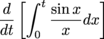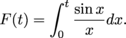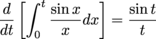Study Guide

# The Fundamental Theorem of Calculus - The Second Fundamental Theorem of Calculus

## The Second Fundamental Theorem of Calculus

As if one Fundamental Theorem of Calculus wasn't enough, there's a second one. The first FTC says how to evaluate the definite integral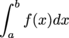if you know an antiderivative of f. The second FTC says how to build an antiderivative of f if you don't know one already.

Given a continuous function f and a constant a, we can define a new function F(x) by integrating f from a to x: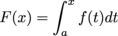We can think of this new function F as an accumulation function—it accumulates the weighted area under f from a to x.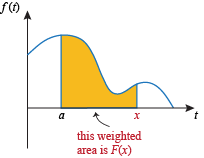Given a continuous function f, we can build a new function F(x) by picking a lower limit of integration a and integrating f from a to x.

The Second Fundamental Theorem of Calculus says that when we build a function this way, we get an antiderivative of f.

### Second Fundamental Theorem of Calculus:

Assume f(x) is a continuous function on the interval I and a is a constant in I. Define a new function F(x) by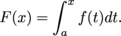Then F(x) is an antiderivative of f(x)—that is, F '(x) = f(x) for all x in I.

That business about the interval I is to make sure we only get limits of integration that are are reasonable for your function. Some things that wouldn't be reasonable:

• Choosing a = -1 for the function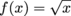. Then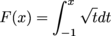doesn't make sense because if x ≠ -1 we're trying to integrate a function over an interval where the function isn't even entirely defined.

• Choosing a = 1 for the function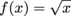but then trying to evaluate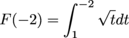Again, we're trying to integrate a function over an interval where the function isn't entirely defined.

• Choosing a = 0 for the function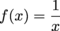. How would you evaluate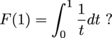The problem is that a = 0 isn't in an interval on which f is continuous. Actually, we can do stuff with this integral—it just requires some extra work, and we haven't gotten there yet.

• Choosing a = -1 for the functionand trying to evaluate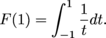If I is any interval that contains both -1 and 1, the function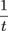isn't continuous on that interval. Again, we can do stuff with this integral, but we haven't learned how yet.

We can condense this discussion into a short warning.

Be Careful: Whenever you go to evaluate an integral, make sure the limits of integration are reasonable for the function you're integrating.

If a function is discontinuous at or between the limits of integration we may still be able to evaluate the integral, but it requires a little extra sneakiness that we haven't gotten to yet.

So far, we've used the second FTC to build antiderivatives for things we already have derivatives for. That's not very exciting. Why go to all the trouble of writing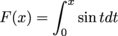to get an antiderivative for sin x, when we already know that

-cos x

is an antiderivative of sin x?

It turns out there are many functions whose antiderivatives we don't know how to find. Some we'll learn how to find using various techniques of integration. However, there are many functions whose antiderivatives can't be written down with a nice formula. We can't write down a nice formula for the antiderivative of

eex

or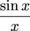,

for example - not just because we don't know how, but because it can't be done.

The second FTC lets us build an antiderivative of f(x) even in the cases where it's impossible to write a nice formula for an antiderivative.

• ### Letters

The second FTC can be confusing with all those letters x and t floating around. Chillax. We're going to straighten them out here.

A function and its derivative use the same independent variable. If we have a function f(x) and we take its derivative, we get a function f '(x). We don't get f '(t).

Working backwards, a function and its antiderivative also use the same independent variable. If we're trying to build an antiderivative for f(x), the antiderivative must also be a function of x. So we need to end up with an antiderivative F(x), not F(t). This is why we use x as a limit of integration: when you change the upper limit of integration, you change the value of the definite integral.

When x is a limit of integration, we don't want to use it as the variable of integration too. Writing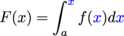is horribly confusing. Other than that, it doesn't matter what letter we use for the variable of integration. We could write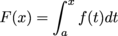or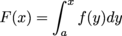or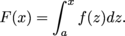When we change the letter, all we're doing is relabeling the horizontal axis. Regardless of the labeling, we're finding the weighted area between f and the horizontal axis from a to x.

• ### Antiderivatives

The FTC tells us how to build antiderivatives for any continuous function we like. No user manuel required. There are some things we need to know about these antiderivatives.

If we define an antiderivative of f(x) by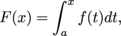then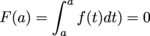because we're integrating over an interval of length 0.

1) If we define two antiderivatives of f(x) by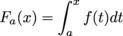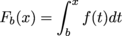where a < b, then the difference between Fb(x) and Fa(x) is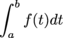which is a constant.

2) Define an antiderivative of f(x) by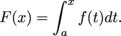Since the derivative of a constant is 0, we can add any constant c that we like to F(x) and get a new antiderivative of f(x). That is, the function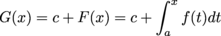has G '(x) = f(x).

Using the information above, we can build antiderivatives with various properties.

• ### Finding Derivatives

The second FTC states that a function of the formis an antiderivative of f(x). This means F '(x) = f(x). Using Leibniz notation,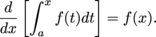### Sample Problem

Find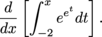The second FTC says that the integral expression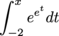is an antiderivative of eex. This means the derivative ofis eex. In Leibniz notation,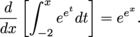In the previous example, all we had to do was write down the integrand, replacing t with x. This works so long as the lower limit of integration is a constant and the upper limit of integration is x all by itself.

We could also change the letters around. If we're using t as the upper limit of integration, then we have an antiderivative F(t) and its derivative will also be a function of t.

### Sample Problem